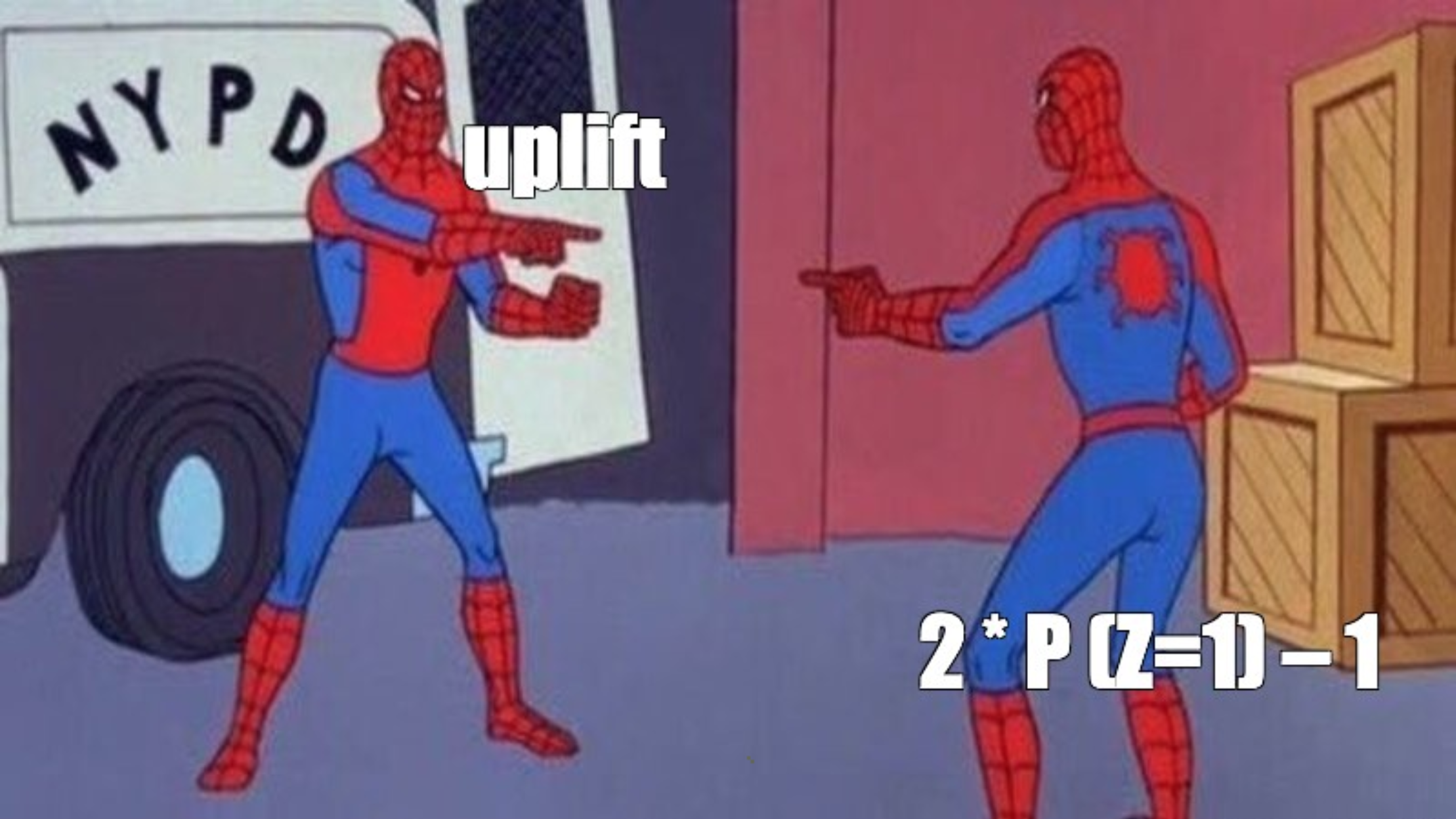# Class Transformation¶

Warning

This approach is only suitable for classification problem

Simple yet powerful and mathematically proven uplift modeling method, presented in 2012. The main idea is to predict a slightly changed target $$Z_i$$:

$Z_i = Y_i \cdot W_i + (1 - Y_i) \cdot (1 - W_i),$
• $$Z_i$$ - a new target for the $$i$$ customer;

• $$Y_i$$ - a previous target for the $$i$$ customer;

• $$W_i$$ - treatment flag assigned to the $$i$$ customer.

In other words, the new target equals 1 if a response in the treatment group is as good as a response in the control group and equals 0 otherwise:

$\begin{split}Z_i = \begin{cases} 1, & \mbox{if } W_i = 1 \mbox{ and } Y_i = 1 \\ 1, & \mbox{if } W_i = 0 \mbox{ and } Y_i = 0 \\ 0, & \mbox{otherwise} \end{cases}\end{split}$

Let’s go deeper and estimate the conditional probability of the target variable:

$\begin{split}P(Z=1|X = x) = \\ = P(Z=1|X = x, W = 1) \cdot P(W = 1|X = x) + \\ + P(Z=1|X = x, W = 0) \cdot P(W = 0|X = x) = \\ = P(Y=1|X = x, W = 1) \cdot P(W = 1|X = x) + \\ + P(Y=0|X = x, W = 0) \cdot P(W = 0|X = x).\end{split}$

We assume that $$W$$ is independent of $$X = x$$ by design. Thus we have: $$P(W | X = x) = P(W)$$ and

$\begin{split}P(Z=1|X = x) = \\ = P^T(Y=1|X = x) \cdot P(W = 1) + \\ + P^C(Y=0|X = x) \cdot P(W = 0)\end{split}$

Also, we assume that $$P(W = 1) = P(W = 0) = \frac{1}{2}$$, which means that during the experiment the control and the treatment groups were divided in equal proportions. Then we get the following:

\begin{align}\begin{aligned}\begin{split}P(Z=1|X = x) = \\ = P^T(Y=1|X = x) \cdot \frac{1}{2} + P^C(Y=0|X = x) \cdot \frac{1}{2} \Rightarrow \\\end{split}\\\begin{split}2 \cdot P(Z=1|X = x) = \\ = P^T(Y=1|X = x) + P^C(Y=0|X = x) = \\ = P^T(Y=1|X = x) + 1 - P^C(Y=1|X = x) \Rightarrow \\ \Rightarrow P^T(Y=1|X = x) - P^C(Y=1|X = x) = \\ = uplift = 2 \cdot P(Z=1|X = x) - 1\end{split}\end{aligned}\end{align}Thus, by doubling the estimate of the new target $$Z$$ and subtracting one we will get an estimation of the uplift:

$uplift = 2 \cdot P(Z=1) - 1$

This approach is based on the assumption: $$P(W = 1) = P(W = 0) = \frac{1}{2}$$. That is the reason that it has to be used only in cases where the number of treated customers (communication) is equal to the number of control customers (no communication).

Hint

In sklift this approach corresponds to the ClassTransformation class.

## Examples using sklift.models.ClassTransformation¶

1. The overview of the basic approaches to the Uplift Modeling problem

1. The 2nd place solution of X5 RetailHero uplift contest by Kirill Liksakov

 In English 🇬🇧 nbviewer github